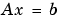MATLAB Function Referencedmperm

Dulmage-Mendelsohn decomposition

Syntax

• ```p = dmperm(A)
[p,q,r,s] = dmperm(A)
```

Description

```p = dmperm(A) ``` if `A` is square and has full rank, returns a row permutation `p` so that `A(p,:)` has nonzero diagonal elements. This permutation is also called a perfect matching. If `A` is not square or not full rank, `p` is a vector that identifies a matching of maximum size: for each column `j` of `A`, either `p(j)=0` or `A(p(j),j)` is nonzero.

```[p,q,r,s] = dmperm(A), ``` where `A` need not be square or full rank, finds permutations p and `q` and index vectors `r` and `s` so that `A(p,q)` is block upper triangular. The `k`th block has indices `(r(k):r(k+1)-1, s(k):s(k+1)-1)`. When `A` is square and has full rank, `r = s`.

If `A` is not square or not full rank, the first block may have more columns and the last block may have more rows. All other blocks are square and irreducible. `dmperm` permutes nonzeros to the diagonals of square blocks, but does not do this for non-square blocks.

Remarks

If `A` is a reducible matrix, the linear systemcan be solved by permuting `A` to a block upper triangular form, with irreducible diagonal blocks, and then performing block backsubstitution. Only the diagonal blocks of the permuted matrix need to be factored, saving fill and arithmetic in the blocks above the diagonal.

In graph theoretic terms, `dmperm` finds a maximum-size matching in the bipartite graph of `A`, and the diagonal blocks of `A(p,q)` correspond to the strong Hall components of that graph. The output of `dmperm` can also be used to find the connected or strongly connected components of an undirected or directed graph. For more information see Pothen and Fan .

See Also

`sprank`

References

  Pothen, Alex and Chin-Ju Fan, "Computing the Block Triangular Form of a Sparse Matrix," ACM Transactions on Mathematical Software, Vol. 16, No. 4, Dec. 1990, pp. 303-324.

© 1994-2005 The MathWorks, Inc.